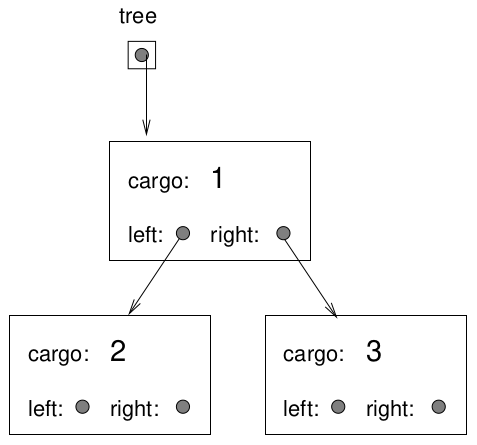# 19. Trees¶

This chapter presents a new abstract data structure called a tree, and discusses some of its uses.

## 19.1. A tree node¶

Like linked lists, trees are made up of nodes. A common kind of tree is a binary tree, in which each node contains a reference to two other nodes (possibly null). We will only be studying binary trees in this book, and we will refer to them as “trees” to simplify our discussion.

The class definition looks like this:

```template <class T>
class Tree {
public:
T cargo;
Tree<T>* left;
Tree<T>* right;

Tree (T cargo, Tree<T>* left, Tree<T>* right) {
this->cargo = cargo;
this->left = left;
this->right = right;
}
};
```

Like linked list nodes, tree nodes contain cargo, which we are again making generic by using a template. The other instance variables, `left` and `right`, are pointers to tree nodes that will be linked together to form a tree.

With this tree node structure defined, we can build a tree by connecting nodes together.

```Tree<int>* tree = new Tree<int>(1, NULL, NULL);
tree->left = new Tree<int>(2, NULL, NULL);
tree->right = new Tree<int>(3, NULL, NULL);
```

We named the pointers `left` and `right` in accordance with the way we represent trees graphically:The top of the tree (the node to which `tree` points) is called the root. In keeping with the tree metaphor, the other nodes are called branches and the nodes at the tips with `NULL` values for both `left` and `right` are called leaves. It may seem odd that we draw the picture with the root at the top and leaves at the bottom, but that is not the strangest thing.

To make things worse, computer scientists mix in yet another metaphor: the family tree. The top node is sometimes called a parent and the nodes it points to are its children. Nodes with the same parent are called siblings, and so on.

Finally, there is also a geometric vocabulary for talking about trees. In addition to “left” and “right”, we refer to “up” (toward the parent/root) and “down” (toward the children/leaves). Also, all the nodes that are the same distance (the number of links from the root) comprise a level of the tree.

## 19.2. A tree is a recursive data structure¶

A recursive data structure is a data structure that is made up of smaller or simpler versions of itself. Recurse data structures can have recursive definition, as in the following:

binary tree
1. An empty tree.

2. A node containing data and two pointers to binary trees.

The first part of this definition provided the base case for the recursion.

Note

Lists are similarly recursive data structures, as their treatment by programming languages like Lisp makes abundantly clear.

Algorithms to manipulate recursive data structures like binary trees tend to lend themselves to the use of recursion, as we will soon see.

## 19.3. Building trees¶

The process of assembling tree nodes is similar to the process of assembling linked list.

## 19.4. Glossary¶

binary tree

A tree in which each node refers to 0, 1, or 2 dependent nodes.

root

The top-most node in a tree, to which no other nodes refer.

leaf

A bottom-most node in a tree, which refers to no other nodes.

parent

The node that refers to a given node.

child

On of the nodes referred to by a node.

level

The set of nodes equidistant from the root.

recurse data structure

A data structure that is partially composed of smaller or simpler examples of itself.

prefix notation

A way of writing a mathematical expression with each operator appearing before its operands.

preorder

A way to traverse a tree, visiting each node before its children.

postorder

A way to traverse a tree, visiting the children of each node before the node itself.

inorder

A way to traverse a tree, visiting the left subtree, then the root, then the right subtree.

binary operator

An operator that takes two operands.